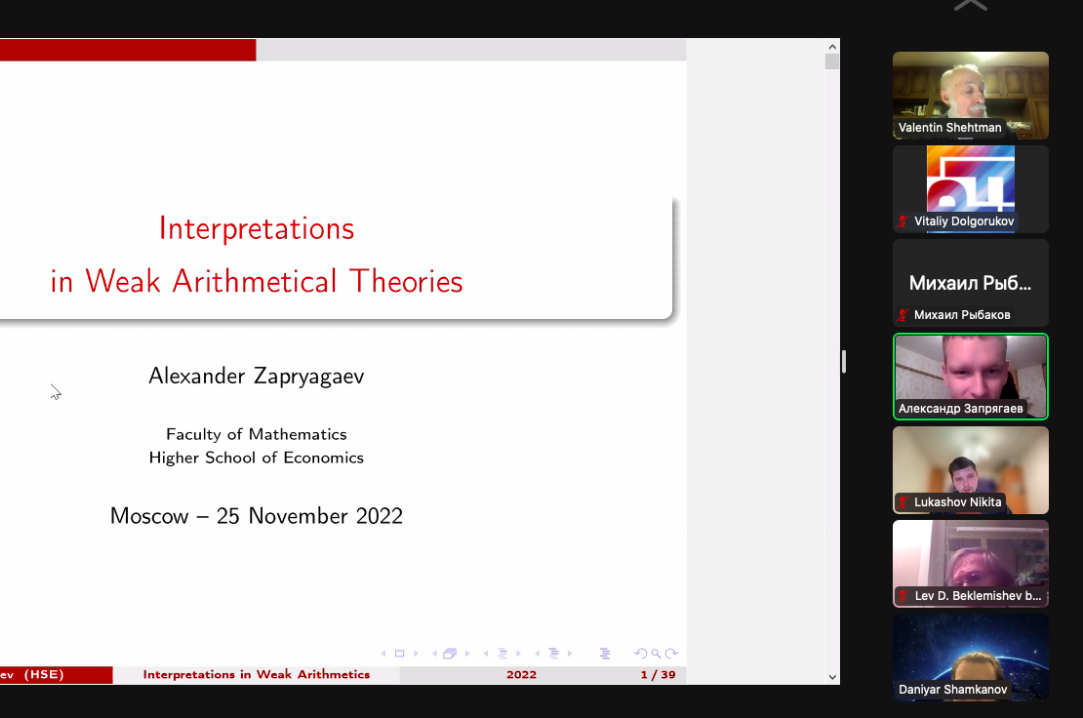• A
• A
• A
• АБВ
• АБВ
• АБВ
• А
• А
• А
• А
• А
Обычная версия сайта

## Международная лаборатория логики, лингвистики и формальной философии

Важные объявления 1

# Прошла предварительная защита кандидатской диссертации Александра Запрягаева "Interpretations of Weak Arithmetical Theories"

25 ноября на заседании научного семинара "Современные проблемы математической логики" в ВШЭ состоялась предварительная защита кандидатской диссертации Александра Запрягаева, написанной под
руководством Льва Беклемишева.Аннотация
Interpretations are a standard tool in model theory and the study of decidability of first-order theories. We consider certain questions on the interpretability of weak arithmetical theories that are well-studied for stronger systems. such as Peano Arithmetic (PA).

We consider non-parametric, one-dimensional or multi-dimensional interpretations of weak arithmetical theories. A. Visser posed the following question : given an weak arithmetical theory T without ability to encode syntax but complete and decidable, does it hold that each interpretation (one-dimensional or multi-dimensional) of T into itself is isomorphic to the trivial one, and, if it is, is the isomorphism always expressible by a formula  of T? (*) He conjectured, in particular, that (*) holds for Presburger Arithmetic PrA, that is, the theory of natural numbers with addition. It is known that PrA is decidable; in fact, it admits quantifier elimination in the extended language L=(=,<,{≡ₙ}). PrA is not finitely axiomatizable.

A family of natural extensions of PrA is given by Büchi arithmetics BAₙ, n ≥ 2, which supplement PrA with an additional unary functional symbol Vₙ(x) denoting the largest power of n that divides x. These theories were introduced by J. Büchi in order to describe the recognizability of sets of natural numbers by finite automata through definability in some arithmetical language. As shown by V. Bruyère, the definability of a set of natural numbers in BAₙ is equivalent to its recognizability by a finite automaton receiving m-tuples of natural numbers in the form of m-tuples of their last, then penultimate, etc. digits of their n-ary expansion. BAₙ are known to be decidable.

As PrA and BAₙ are the true theories of their respective standard models, it suffices to consider the interpretations into the standard model.

In the thesis, the author obtains the property (*) for Presburger Arithmetic in both one-dimensional and multi-dimensional cases, thus resolving Visser's conjecture. The following theorem is obtained in the joint publication with F. Pakhomov :

Theorem 1. (1) For each interpretation of PrA in (ℕ;=,+), the internal model is standard;
(2) The isomorphism between the internal model and ℕ can be expressed by a PrA-formula.

Note that Theorem 1 (1) in the one-dimensional case was previously established by Zoethout . In order to show (1), a rank-based necessary condition for linear orders to be m-dimensionally interpretable in Presburger arithmetic is given. Let the rank of a linear order L be the minimal ordinal α such that α iterations of identifying the points on finite distance from each other lead to a finite order, or infinity in case it is impossible. Then the following holds:

Theorem 2. All linear orders m-dimensionally interpretable in (ℕ;=,+) have rank m or below.

In particular, as ℕ + ℤ · ℚ is the order-type of all countable non-standard models of PrA, and its rank is infinity, (1) is established.

In order to show an isomorphism between the internal model and external ℕ is always definable, a description is given of sets definable in Presburger arithmetic. A theory of piecewise polynomial functions is developed, which correspond to functions giving the cardinality of sections of Presburger-definable sets. The result of Theorem 1 (2) follows by growth rate considerations.

In the case of Büchi arithmetics, the following result is established:

Theorem 3. For each interpretation of BAₙ in (ℕ;=,+,Vₙ), the internal model is standard.

We note that if BAₙ is interpreted in BAₙ by interpretation ι, then it can be extended to an interpretation of (ℤ, +, <) such that the internal model of the original theory will correspond to the non-negative numbers. This is an interpretation of an abelian group (Z,+). A contradiction is reached between a condition on automatic torsion-free abelian groups found by Braun and Strüngmann and the description of the order types of non-standard models of Büchi arithmetics.

Whether the isomorphism given by Theorem 3 is always definable remains an open problem.

 F. Pakhomov and A. Zapryagaev. Multi-dimensional interpretations of Presburger arithmetic in itself. Journal of Logic and Computation, 30.8, 1681–1693, 2020

 A. Visser. Friedman-reflexivity. Annals of Pure and Applied Logic, 173(9), 103-160, 2022

 J. Zoethout. Interpretations in Presburger Arithmetic. BS Thesis, Utrecht University, 2015